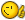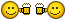# Convert Ellipse to Arc## Recommended PostsI have been trying to figure this out for a couple of days now. The only thing I could find is this LISP that takes the Ellipse properties and uses them to creat an arc.

When I use this, the start and end angles are not correct in the drawing. Ellipses must have been drawn in a different UCS).

I have tried to get the LISP to use the start and end points of the ellipse along with the radius and center point. For the life of me I cannot figure it out. If anyone knows how to do this please let me know.

*NOTE* Elllipses that I am trying to convert have the same minor and major radii.

` (defun c:e2a (/ acaddoc acadms acadobj center endangle obj radius ss ssn startangle)  (vl-load-com)  (if     (setq ss (ssget '((0 . "ellipse"))))    (progn            (setq acadobj (vlax-get-acad-object))      (setq acaddoc (vla-get-activeDocument acadobj))      (setq acadms (vla-get-modelspace acaddoc))      (setq ssn (ssname ss 0))      (setq obj (vlax-ename->vla-object ssn))      (if obj ;(equal (vla-get-RadiusRatio obj) 1 0.0001) (progn   (setq radius (vla-get-MajorRadius obj))   (setq Startangle (vla-get-Startangle obj))   (setq Endangle (vla-get-Endangle obj))   (setq Center (vlax-get obj 'center))   (entdel ssn)   (vla-addarc acadms (vlax-3d-point Center) radius Startangle Endangle)   ) ; progn (alert "> Ellipse objects failed to be converted") )   ; if      )     ; progn    )       ; if  (princ)  )       ; defun[/Code]`
##### Share on other sitesHi Bill,

I would be inclined to use the entmake(x) function for this task, as transference of object properties can be accomplished with a single function call.

Here is my program:

```[color=GREEN];; Ellipse 2 Arc  -  Lee Mac 2011  -  www.lee-mac.com[/color]
[color=GREEN];; Converts Circular Ellipses (Axis Ratio = 1) to Circles/Arcs[/color]

([color=BLUE]defun[/color] c:e2a ( [color=BLUE]/[/color] e i s )
([color=BLUE]if[/color] ([color=BLUE]setq[/color] s ([color=BLUE]ssget[/color] [color=MAROON]"_:L"[/color] '((0 . [color=MAROON]"ELLIPSE"[/color]) (40 . 1.0))))
([color=BLUE]repeat[/color] ([color=BLUE]setq[/color] i ([color=BLUE]sslength[/color] s))
([color=BLUE]setq[/color] e ([color=BLUE]entget[/color] ([color=BLUE]ssname[/color] s ([color=BLUE]setq[/color] i ([color=BLUE]1-[/color] i)))))
([color=BLUE]if[/color]
([color=BLUE]entmakex[/color]
([color=BLUE]if[/color] ([color=BLUE]equal[/color] ([color=BLUE]abs[/color] ([color=BLUE]-[/color] ([color=BLUE]cdr[/color] ([color=BLUE]assoc[/color] 42 e)) ([color=BLUE]cdr[/color] ([color=BLUE]assoc[/color] 41 e)))) ([color=BLUE]+[/color] [color=BLUE]pi[/color] [color=BLUE]pi[/color]))
([color=BLUE]apply[/color] '[color=BLUE]append[/color]
([color=BLUE]cons[/color]
([color=BLUE]list[/color]
([color=BLUE]cons[/color] 0 [color=MAROON]"CIRCLE"[/color])
([color=BLUE]cond[/color] (([color=BLUE]assoc[/color]  6 e)) ('( 6 . [color=MAROON]"BYLAYER"[/color])))
([color=BLUE]cond[/color] (([color=BLUE]assoc[/color] 39 e)) ('(39 . 0.0)))
([color=BLUE]cond[/color] (([color=BLUE]assoc[/color] 62 e)) ('(62 . 256)))
([color=BLUE]cons[/color] 10 ([color=BLUE]trans[/color] ([color=BLUE]cdr[/color] ([color=BLUE]assoc[/color] 10 e)) 0 ([color=BLUE]cdr[/color] ([color=BLUE]assoc[/color] 210 e))))
([color=BLUE]cons[/color] 40 ([color=BLUE]distance[/color] '(0. 0. 0.) ([color=BLUE]cdr[/color] ([color=BLUE]assoc[/color] 11 e))))
)
([color=BLUE]mapcar[/color]
([color=BLUE]function[/color]
([color=BLUE]lambda[/color] ( x )
([color=BLUE]if[/color] ([color=BLUE]not[/color] ([color=BLUE]member[/color] ([color=BLUE]car[/color] x) '(-1 0 5 6 10 11 39 40 41 42 62 100 330)))
([color=BLUE]list[/color] x)
)
)
)
e
)
)
)
([color=BLUE]apply[/color] '[color=BLUE]append[/color]
([color=BLUE]cons[/color]
([color=BLUE]list[/color]
([color=BLUE]cons[/color] 0 [color=MAROON]"ARC"[/color])
([color=BLUE]cond[/color] (([color=BLUE]assoc[/color]  6 e)) ('( 6 . [color=MAROON]"BYLAYER"[/color])))
([color=BLUE]cond[/color] (([color=BLUE]assoc[/color] 39 e)) ('(39 . 0.0)))
([color=BLUE]cond[/color] (([color=BLUE]assoc[/color] 62 e)) ('(62 . 256)))
([color=BLUE]cons[/color] 10 ([color=BLUE]trans[/color] ([color=BLUE]cdr[/color] ([color=BLUE]assoc[/color] 10 e)) 0 ([color=BLUE]cdr[/color] ([color=BLUE]assoc[/color] 210 e))))
([color=BLUE]cons[/color] 40 ([color=BLUE]distance[/color] '(0. 0. 0.) ([color=BLUE]cdr[/color] ([color=BLUE]assoc[/color] 11 e))))
([color=BLUE]cons[/color] 50 ([color=BLUE]+[/color] ([color=BLUE]cdr[/color] ([color=BLUE]assoc[/color] 41 e)) ([color=BLUE]angle[/color] '(0. 0. 0.) ([color=BLUE]trans[/color] ([color=BLUE]cdr[/color] ([color=BLUE]assoc[/color] 11 e)) 0 1))))
([color=BLUE]cons[/color] 51 ([color=BLUE]+[/color] ([color=BLUE]cdr[/color] ([color=BLUE]assoc[/color] 42 e)) ([color=BLUE]angle[/color] '(0. 0. 0.) ([color=BLUE]trans[/color] ([color=BLUE]cdr[/color] ([color=BLUE]assoc[/color] 11 e)) 0 1))))
)
([color=BLUE]mapcar[/color]
([color=BLUE]function[/color]
([color=BLUE]lambda[/color] ( x )
([color=BLUE]if[/color] ([color=BLUE]not[/color] ([color=BLUE]member[/color] ([color=BLUE]car[/color] x) '(-1 0 5 6 10 11 39 40 41 42 62 100 330)))
([color=BLUE]list[/color] x)
)
)
)
e
)
)
)
)
)
([color=BLUE]entdel[/color] ([color=BLUE]cdr[/color] ([color=BLUE]assoc[/color] -1 e)))
)
)
)
([color=BLUE]princ[/color])
)```

Edited by Lee Mac
##### Share on other sitesI tried your program, and it ends up doing the same as what I had. It replaces the ellipse with an arc based on the start and end angles, causeing the arc to be created in a different location than where the ellipse currently is. The properties of the new arc and the old Ellipse have the same start and end angles, but the arc is drawn as if it was rotated. I hope that makes sense.

If the program could use the start and end points instead of the angles, I think it would work.

##### Share on other sitesHi Bill,

I missed something, try the updated code above##### Share on other sitesLee Mac you are a genius! It works amazing. I cant thank you enough. You saved me lots time on here.

Thanks again!!

##### Share on other sitesThanks Bill, you're welcome##### Share on other sites

• 3 years later...Just started using this lisp in 2014.

For some reason it will not select any ellipse. I suspect it has to do with how 2014 creates the ellipse.

Wonder if anyone else has encountered this, or if they have a different way to convert ellipses into arcs?

##### Share on other sitesFor some reason it will not select any ellipse. I suspect it has to do with how 2014 creates the ellipse.

Could you upload a sample drawing?

##### Share on other sitesFor some reason it will not select any ellipse. I suspect it has to do with how 2014 creates the ellipse.

Lee's code is fine, but I suspect that you are attempting to select real ellipse and not circular one - in selection filter that follows (ssget) function it was explicitly written that ratio between "small" and "big" ellipse radius is 1.0 which means that ellipse must be circular in order for (ssget) function to accept selecting entities filtered by written filter statement that follows inside (ssget) function...

##### Share on other sitesPerhaps it may be some setting I have set.

The ellipse are circular with a radius ratio of 1.

Attached is a drawing that will not allow me to select the ellipses with the lisp.

Ellipse.dwg

##### Share on other sitesThe ellipse are circular with a radius ratio of 1.

Attached is a drawing that will not allow me to select the ellipses with the lisp.

Thank you for attaching the sample drawing.

The issue is that, although very close to being circular, the ellipse axis ratio is in fact not quite equal to 1.0.

You can verify this by using the following program to retrieve the axis ratio for a selected ellipse:

```(defun c:eratio ( / e )
(while
(and (setq e (car (entsel "\nSelect ellipse: ")))
(= "ELLIPSE" (cdr (assoc 0 (entget e))))
)
(princ (strcat "\nEllipse ratio: " (rtos (cdr (assoc 40 (entget e))) 2 15)))
)
(princ)
)```

Testing the program with a few ellipses from your drawing yields the following results:

```Select ellipse:
Ellipse ratio: 0.999999532781626
Select ellipse:
Ellipse ratio: 0.999999532795758
Select ellipse:
Ellipse ratio: 0.999999532786339
Select ellipse:
Ellipse ratio: 0.999999532749180
Select ellipse:
Ellipse ratio: 0.999999532857222
```

The following code is a modification of the Ellipse to Arc program published on my site here, to incorporate some tolerance into the ellipse ratio filter:

```[color=GREEN];;-------------------=={ Ellipse to Arc }==-------------------;;[/color]
[color=GREEN];;                                                            ;;[/color]
[color=GREEN];;  Converts circular Ellipses & Elliptical Arcs (i.e. for    ;;[/color]
[color=GREEN];;  which the Ellipse Axis Ratio = 1.0) to Circles & Arcs,    ;;[/color]
[color=GREEN];;  whilst retaining all original properties. Works with      ;;[/color]
[color=GREEN];;  Ellipses & Elliptical Arcs constructed in any UCS.        ;;[/color]
[color=GREEN];;------------------------------------------------------------;;[/color]
[color=GREEN];;------------------------------------------------------------;;[/color]

([color=BLUE]defun[/color] c:e2a ( [color=BLUE]/[/color] a b c e i m p q r s u v z )
([color=BLUE]if[/color] ([color=BLUE]setq[/color] s ([color=BLUE]ssget[/color] [color=MAROON]"_:L"[/color] '((0 . [color=MAROON]"ELLIPSE"[/color]) (-4 . [color=MAROON]">="[/color]) (40 . 0.9999) (-4 . [color=MAROON]"<="[/color]) (40 . 1.0001))))
([color=BLUE]repeat[/color] ([color=BLUE]setq[/color] i ([color=BLUE]sslength[/color] s))
([color=BLUE]setq[/color] e ([color=BLUE]entget[/color] ([color=BLUE]ssname[/color] s ([color=BLUE]setq[/color] i ([color=BLUE]1-[/color] i))))
z ([color=BLUE]cdr[/color] ([color=BLUE]assoc[/color] 210 e))
c ([color=BLUE]trans[/color] ([color=BLUE]cdr[/color] ([color=BLUE]assoc[/color] 10 e)) 0 z)
p ([color=BLUE]trans[/color] ([color=BLUE]cdr[/color] ([color=BLUE]assoc[/color] 11 e)) 0 z)
a ([color=BLUE]distance[/color] '(0.0 0.0) p)
b ([color=BLUE]*[/color] a ([color=BLUE]cdr[/color] ([color=BLUE]assoc[/color] 40 e)))
r ([color=BLUE]angle[/color] '(0.0 0.0) p)
u ([color=BLUE]cdr[/color] ([color=BLUE]assoc[/color] 41 e))
v ([color=BLUE]cdr[/color] ([color=BLUE]assoc[/color] 42 e))
m ([color=BLUE]list[/color] ([color=BLUE]list[/color] ([color=BLUE]cos[/color] r) ([color=BLUE]-[/color] ([color=BLUE]sin[/color] r))) ([color=BLUE]list[/color] ([color=BLUE]sin[/color] r) ([color=BLUE]cos[/color] r)))
p ([color=BLUE]mapcar[/color] '[color=BLUE]+[/color] c (mxv m ([color=BLUE]list[/color] ([color=BLUE]*[/color] a ([color=BLUE]cos[/color] u)) ([color=BLUE]*[/color] b ([color=BLUE]sin[/color] u)))))
q ([color=BLUE]mapcar[/color] '[color=BLUE]+[/color] c (mxv m ([color=BLUE]list[/color] ([color=BLUE]*[/color] a ([color=BLUE]cos[/color] v)) ([color=BLUE]*[/color] b ([color=BLUE]sin[/color] v)))))
)
([color=BLUE]if[/color]
([color=BLUE]if[/color] ([color=BLUE]equal[/color] p q 1e-
([color=BLUE]entmake[/color]
([color=BLUE]cons[/color] '(0 . [color=MAROON]"CIRCLE"[/color])
([color=BLUE]append[/color] (LM:defaultprops e)
([color=BLUE]list[/color]
([color=BLUE]cons[/color]  010 c)
([color=BLUE]cons[/color]  040 a)
([color=BLUE]assoc[/color] 210 e)
)
)
)
)
([color=BLUE]entmake[/color]
([color=BLUE]cons[/color] '(0 . [color=MAROON]"ARC"[/color])
([color=BLUE]append[/color] (LM:defaultprops e)
([color=BLUE]list[/color]
([color=BLUE]cons[/color]  010 c)
([color=BLUE]cons[/color]  040 a)
([color=BLUE]cons[/color]  050 ([color=BLUE]angle[/color] c p))
([color=BLUE]cons[/color]  051 ([color=BLUE]angle[/color] c q))
([color=BLUE]assoc[/color] 210 e)
)
)
)
)
)
([color=BLUE]entdel[/color] ([color=BLUE]cdr[/color] ([color=BLUE]assoc[/color] -1 e)))
)
)
)
([color=BLUE]princ[/color])
)

[color=GREEN];; Default Properties  -  Lee Mac[/color]
[color=GREEN];; Returns a list of DXF properties for the supplied DXF data,[/color]
[color=GREEN];; substituting default values for absent DXF groups[/color]

([color=BLUE]defun[/color] LM:defaultprops ( elist )
([color=BLUE]mapcar[/color]
([color=BLUE]function[/color]
([color=BLUE]lambda[/color] ( pair )
([color=BLUE]cond[/color] (([color=BLUE]assoc[/color] ([color=BLUE]car[/color] pair) elist)) ( pair ))
)
)
'(
(008 . [color=MAROON]"0"[/color])
(006 . [color=MAROON]"BYLAYER"[/color])
(039 . 0.0)
(062 . 256)
(048 . 1.0)
(370 . -1)
)
)
)

[color=GREEN];; Matrix x Vector  -  Vladimir Nesterovsky[/color]
[color=GREEN];; Args: m - nxn matrix, v - vector in R^n[/color]

([color=BLUE]defun[/color] mxv ( m v )
([color=BLUE]mapcar[/color] '([color=BLUE]lambda[/color] ( r ) ([color=BLUE]apply[/color] '[color=BLUE]+[/color] ([color=BLUE]mapcar[/color] '[color=BLUE]*[/color] r v))) m)
)

([color=BLUE]princ[/color])
```

##### Share on other sitesNever thought of checking the ratio units that far.

Perfect. Thanks Lee Mac.

##### Share on other sitesYou're welcome Bill##### Share on other sitesI agree with Bill.... the program is not recognizing the ellipse.... so it is not getting selected.

i understand this is an old thread..... maybe the forum has shifted to another thread... it will be useful to convert ellipses to mathematically accurate circles / arcs##### Share on other sitesI agree with Bill.... the program is not recognizing the ellipse.... so it is not getting selected.

What is the axis ratio of your ellipses? (use the above program to check this)

##### Share on other sitesI have now updated the Ellipse to Arc program published on my site to incorporate the tolerance on the ellipse axis ratio.

Lee

##### Share on other sites

• 2 years later...hi everyone,

Helal-Fl-26-D2.dwg

##### Share on other sitesRefer to the solution offered here.

## Join the conversation

You can post now and register later. If you have an account, sign in now to post with your account.
Note: Your post will require moderator approval before it will be visible.×   Pasted as rich text.   Restore formatting

Only 75 emoji are allowed.